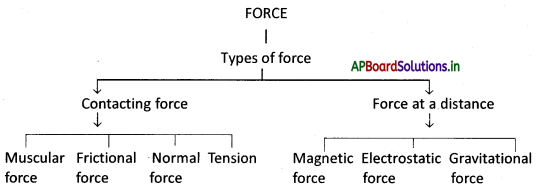# AP Board 8th Class Physical Science Notes Chapter 1 Force

Students can go through AP State Board 8th Class Physical Science Notes Chapter 1 Force to understand and remember the concept easily.

## AP State Board Syllabus 8th Class Physical Science Notes Chapter 1 Force

→ Force is a push or a pull.

→ A force can act on an object with or without being in contact with it.

→ A force acting on the body is either a contact force or a force at a distance.

→ A direction that is perpendicular to the plane of a surface is said to be normal.

→ The force that a solid surface exerts on any object in the normal direction is called the normal force.

→ Tension always pulls the objects along the string.

→ The region in which an object at a point experiences force exerted by another object is called field.

→ The attractive force between any two massive objects is called gravitational force.

→ The force exerted by a magnet on another magnet is called magnetic force.

→ The magnetic force also acts on magnetic material such as iron, nickel.

→ The force exerted by a charged body on another charged or uncharged body is known as electrostatic force.

→ Friction is the force that opposes the relative motion of the surface in contact.

→ The force which we exert by using our body muscles is known as muscular force.

→ Force has magnitude as well as direction.

→ A change in the speed of the object or the direction of its motion or both implies a change in its state of motion.

→ The algebraic sum of all forces acting on a body is known as net force and is denoted before

→ A force can change the state of motion of an object.→ Force may cause a change in the shape of an object.

→ The force acting on the unit area of a surface is called pressure

→ Force: A push or a pull on an object is called a force.

→ Push: Move forward by using force.

→ Pull: Apply force so as to move it towards oneself or the origin of the force.

→ Contact force: The force existing between two interacting objects is called contact force.

→ Force at a distance: The force which acts between two bodies that are not directly touching each other is called force at a distance.

→ Field: Field ¡s a region ¡n which a force can be experienced by another object placed at any point ¡n that region.

→ Friction: The resistance to the movement of a body over the surface of another body.

→ Muscular force: The force exerted by body muscles is coiled muscular force.

→ Gravitational force: The force which pulls the object towards the earth is called gravitational force or force of gravity.

→ Magnetic force: The force exerted by o magnet on another magnet is called magnetic force.

→ Electrostatic force: The force exerted by a charged body on another charged or uncharged body is known as electrostatic force.→ Net force: The algebraic sum of all the forces acting on a body is known as the net force.

→ Magnitude: The size of a quantity is called magnitude.

→ Equilibrium: A state in which opposing forces are balanced.

→ Normal force: The force that a solid force exerts on any object in the normal direction ¡s called the normal force.

→ Tension: The tightness of rope or string when it is stretched is called tension.

→ State of motion: A change ¡n the speed of an object or the direction of its motion or both is called its state of motion.

→ Pressure: The force acting perpendicularly on a unit area of a surface is called pressure.

→ Free body diagram: The diagram showing all the forces acting on an object at a particular instant is called the free body diagram.

→ Magnetic field: The space around the magnet where its influence can be detected s called the magnetic field→ Sir Isaac Newton:

• A British Physicist and Mathematician of high repute studied the color and composition of white light
• His studies on planetary motion led to his famous universal law of gravitation.
• He formulated three fundamental laws of mechanics called Newton‘s laws of motion.
• His main works are Principia and optics.